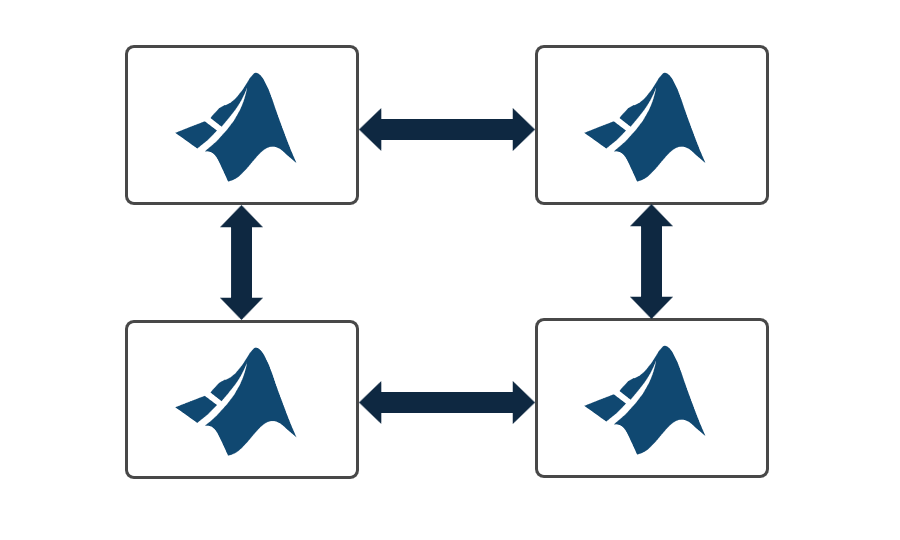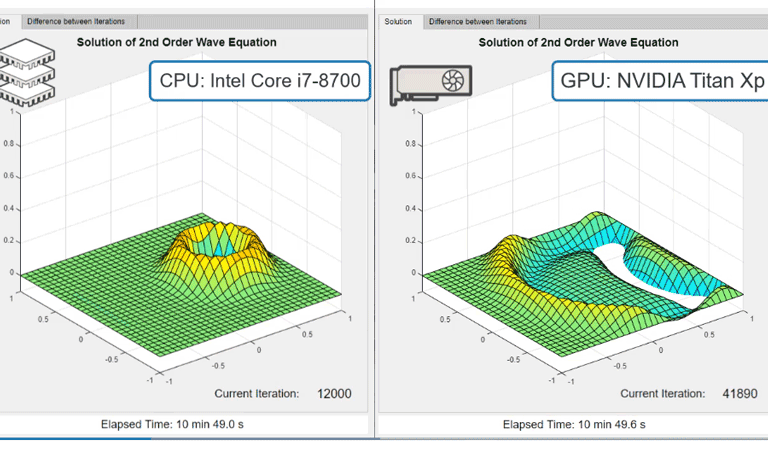# Get Started with Parallel Computing Toolbox

Perform parallel computations on multicore computers, GPUs, and computer clusters

Parallel Computing Toolbox™ lets you solve computationally and data-intensive problems using multicore processors, GPUs, and computer clusters. High-level constructs—parallel for-loops, special array types, and parallelized numerical algorithms—enable you to parallelize MATLAB® applications without CUDA or MPI programming. The toolbox lets you use parallel-enabled functions in MATLAB and other toolboxes. You can use the toolbox with Simulink® to run multiple simulations of a model in parallel. Programs and models can run in both interactive and batch modes.

The toolbox lets you use the full processing power of multicore desktops by executing applications on workers (MATLAB computational engines) that run locally. Without changing the code, you can run the same applications on clusters or clouds (using MATLAB Parallel Server™). You can also use the toolbox with MATLAB Parallel Server to execute matrix calculations that are too large to fit into the memory of a single machine.

## VideosParallel Computing Toolbox Overview
Scale up your computations in parallel on multicore computers, GPUs, and clustersIntroduction to GPU Computing with MATLAB
Accelerate your MATLAB applications with GPUs and built-in GPU support

## Support